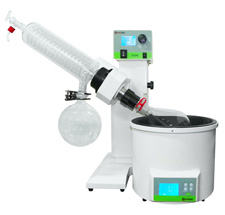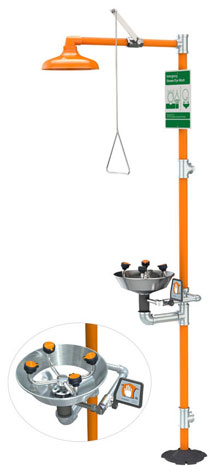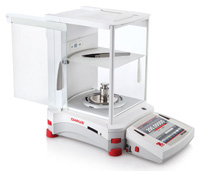MSDSTopics Free Sites FAQ's Regulations Glossary Software Suppliers Books Forum Poll Fun stuff Quiz Store Understand your MSDS with the MS-Demystifier Search ALL our MSDS infoSafety Emporium has lab equipment you need such as rotary evaporators.

Concentration Units

### Definition

The concentration of a chemical substance expresses the amount of a substance present in a mixture. There are many different ways to express concentration.

Chemists use the term solute to describe the substance of interest and the term solvent to describe the material in which the solute is dissolved. For example, in a can of soda pop (a solution of sugar in carbonated water), there are approximately twelve tablespoons of sugar (the solute) dissolved in the carbonated water (the solvent). In general, the component that is present in the greatest amount is termed the solvent.

There are many ways to express concentrations. Some of the more common concentration units are:

1. Mass per unit volume. Some SDS's use milligrams per milliliter (mg/mL) or milligrams per cubic centimeter (mg/cm3). Note that 1 mL = 1 cm3 and that cm3 is sometimes denoted as a "cc" (see volume units and mass units).

Mass per unit volume is handy when discussing how soluble a material is in water or a particular solvent. For example, "the solubility of substance X is 3 grams per liter".

2. Percent by mass. Also called weight percent or percent by weight, this is simply the mass of the solute divided by the total mass of the solution and multiplied by 100%:

$$\mathsf{Percent\,by\,mass = }\left(\frac{\mathsf{Mass\,of\,component}}{\mathsf{Mass\,of\,solution}}\right) \mathsf{(100\%)}$$

The mass of the solution is equal to the mass of the solute plus the mass of the solvent. For example, a solution consisting of 30 grams of sodium chloride and 70 grams of water would be 30% sodium chloride by mass:

$$\mathsf{Percent\,by\,mass = }\left(\frac{\mathsf{30\,g\,NaCl}}{\mathsf{30\,g\,NaCl\,+\,70\,g\,water}}\right) \mathsf{(100\%)=30\%}$$

To avoid confusion whether a solution is percent by weight or percent by volume, the symbol "w/w" (for weight to weight) is often used after the concentration such as "10% potassium iodide solution in water (w/w)".

3.Work safely in the lab with eye/face washes, safety showers, drench hoses and more from Safety Emporium.

4. Percent by volume. Also called volume percent or percent by volume, this is typically only used for mixtures of liquids. Percent by volume is simply the volume of the solute divided by the sum of the volumes of the other components multiplied by 100%:

$$\mathsf{Percent\,by\,volume = }\left(\frac{\mathsf{Volume\,of\,component}}{\mathsf{Volume\,of\,component + volume\,of\,solvent}}\right) \mathsf{(100\%)}$$

If we mix 30 mL of ethanol and 70 mL of water, the percent ethanol by volume will be 30% BUT the total volume of the solution will NOT be 100 mL (although it will be close). That's because ethanol and water molecules interact differently with each other than they do with themselves.

To avoid confusion whether we have a percent by weight or percent by volume solution, we could label this as "30% ethanol in water (v/v)" where v/v stands for "volume to volume".

5. Molarity. Molarity is the number of moles of solute dissolved in one liter of solution. For example, if we have 90 grams of glucose (molar mass = 180 grams per mole) this corresponds to 0.50 moles of glucose:

$$\mathsf{\#\,of\,moles\,of\,solute = }\left(\frac{\mathsf{90\,g}}{\mathsf{180\,g/mol}}\right)= \mathsf{0.5\,mol}$$

If we place 0.5 mol of glucose in a flask and add water until the total volume = 1 liter (1 L) we would have a 0.5 molar solution. Molarity is usually denoted with an italicized capital M, i.e., a 0.50 M solution or with units of mol/L:

$$\mathsf{Molarity = }\left(\frac{\mathsf{0.5\,mol}}{\mathsf{1.0\,L}}\right)= \mathsf{0.5\,}M=\mathsf{0.5\,mol/L}$$

Recognize that molarity is moles of solute per liter of solution, not per liter of solvent!! Also recognize that molarity changes slightly with temperature because the volume of a solution changes with temperature.

6. Normality. Normality is very similar to molarity, however instead of being the the number of moles of solute dissolved in one liter of solution, it reflects the number of mole equivalents per liter of solution. The units are given as eq/L or an italicized capital "N" as in 1.4 N.

Normality is generally only used when a substance has more than one sub-species that can participate in a specified reaction such as a proton for acid/base reactions, an electron for oxidation/reduction reactions, or in precipitation reactions. For example, sulfuric acid has two ionizable protons (H+) which can participate in the neutralization of a base such as sodium hydroxide, NaOH:

H2SO4(aq) + 2 NaOH   →   2 Na+(aq) + 2 H2O + SO42+(aq)

So if the concentration of our sulfuric acid solution is 1 M (mol/l) expressed as a molarity then it is 2 N expressed as normality. We call these solutions 1 molar and 2 normal, respectively.

7. Molality. Molality is the number of moles of solute dissolved in one kilogram of solvent. Notice the two key differences between molarity and molality. Molality uses mass rather than volume and uses solvent instead of solution.

Unlike molarity, molality is independent of temperature because mass does not change with temperature. If we were to place 90 grams of glucose (0.50 moles) in a flask and then add one kilogram of water we would have a 0.50 molal solution:

$$\mathsf{Molality = }\left(\frac{\mathsf{0.5\,mol}}{\mathsf{1.0\,kg}}\right)= \mathsf{0.5\,}m=\mathsf{0.5\,mol/kg}$$

Molality is usually denoted with a small italicized m, i.e. a 0.50 m solution. Note: m also has other possible meanings on Safety Data Sheets, so look at the context carefully.

8. Parts per million (ppm). Parts per million works like percent by mass, but is more convenient when there is only a small amount of solute present. PPM is defined as the mass of the component in solution divided by the total mass of the solution multiplied by 106 (one million):

$$\mathsf{Parts\,per\,million = }\left(\frac{\mathsf{Mass\,of\,component}}{\mathsf{Mass\,of\,solution}}\right) \mathsf{(1{,}000{,}000)}$$

A solution with a concentration of 1 ppm has 1 gram of substance for every million grams of solution. Because the density of water is 1 g per mL and we are adding such a tiny amount of solute, the density of a solution at such a low concentration is approximately 1 g per mL. Therefore, in general, one ppm implies one milligram of solute per liter of solution.

Finally, recognize that one percent = 10,000 ppm. Therefore, something that has a concentration of 300 ppm could also be said to have a concentration of (300 ppm)/(10{,}000 ppm/percent) = 0.03% percent by mass.

9. Parts per billion (ppb) This works like above, but we multiply by one billion (109:

$$\mathsf{Parts\,per\,billion = }\left(\frac{\mathsf{Mass\,of\,component}}{\mathsf{Mass\,of\,solution}}\right) \mathsf{(1{,}000{,}000{,}000)}$$

Caution: the word billion has different meanings in different countries. A solution with 1 ppb of solute has 1 microgram (10-6 g) of material per liter.

10. Parts per trillion (ppt). This works like parts per million and parts per billion except that we multiply by one trillion (1012):

$$\mathsf{Parts\,per\,trillion = }\left(\frac{\mathsf{Mass\,of\,component}}{\mathsf{Mass\,of\,solution}}\right) \mathsf{(1{,}000{,}000{,}000{,}000)}$$

There are few, if any, solutes which are harmful at concentrations as low as 1 ppt. Note: "ppt" is sometimes used as laboratory shorthand for precipitate, which is entirely unrelated.

Here is a handy conversion calculator for several of these terms. Conversions between the other units require knowing additional information and are therefore more complex.

 Concentration Conversion Calculator(Enter number on the left side; answer appears on the right side) This many: Percent by massParts per millionParts per billionParts per trillion Equals Percent by massParts per millionParts per billionParts per trillionLaboratory operations are a breeze with Ohaus analytical balances from Safety Emporium.

### SDS Relevance

Consult the Safety Data Sheets for the chemicals you work with so you know that concentrations could be dangerous or lethal. Always minimize your exposure to chemicals and work within the permissible exposure limits for each substance. Use appropriate administrative and engineering controls to prevent exposures in the first place and personal protective equipment if necessary.